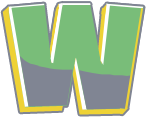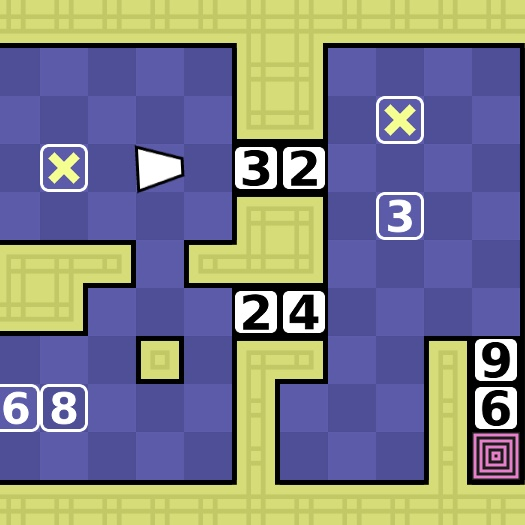Math Push
Math Push is an interesting puzzle game. You have to push blocks filled with numbers around in order to create equations matching numbers.Math Push
6 3.25
Fullscreen

## Recommended for you

Wordle Unlimited» Math Push

# Math Push

Share

Math Push is an interesting puzzle game. You have to push blocks filled with numbers around in order to create equations matching numbers. These numbers will disappear once you've completed the equation. This will allow you to move on to the next level. Although it seems simple at the beginning, it gets more difficult. Add numbers blocks to reach the sum required to complete the level. This fun combination of Sokoban and math puzzle games is what you will find in this game. Have fun!

Rob1221 developed Math Push.

Comment (0)Be the first to comment